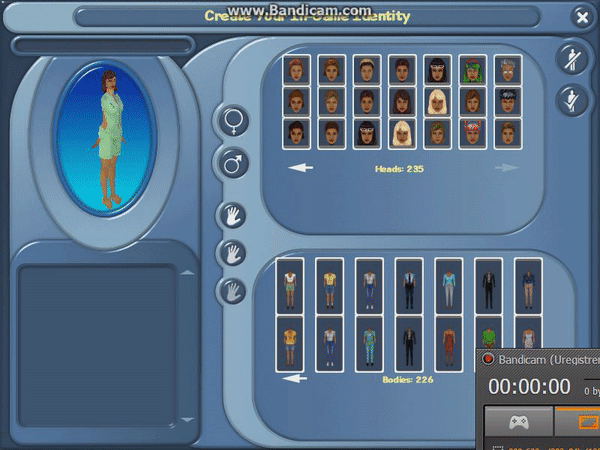-=+=- -=+=- -=+=- -=+=- -=+=- -=+=- -=+=- -=+=- -=+=- -=+=- -=+=- -=+=- -=+=- -=+=- -=+=- -=+=- -=+=- -=+=- -=+=- -=+=- -=+=- -=+=- -=+=- -=+=- -=+=- -=+=- -=+=- -=+=- -=+=- -=+=- (c) WidthPadding Industries 1987 0|150|0 -=+=- -=+=- -=+=- -=+=- -=+=- -=+=- -=+=- -=+=- -=+=- -=+=- -=+=- -=+=- -=+=- -=+=- -=+=- -=+=- -=+=- -=+=- -=+=- -=+=- -=+=- -=+=- -=+=- -=+=- -=+=- -=+=- -=+=- -=+=- -=+=- -=+=-
Socoder -> C/C++/C#/Other -> Shader woes

Sun, 13 Aug 2017, 04:55
Afr0I'm guessing that noone here are particularly good with shaders (specifically: HLSL), but in case you are, can you spot what's wrong with this?

All the bones keep rotating around the X axis when they're only supposed to rotate around the Y.Here's how I create the rotation:

-=-=-
Afr0 Games

Project Dollhouse on Github - Please fork!Sun, 13 Aug 2017, 05:08
JayenkaiI know nothing of shaders, or 3D in general I'm afraid.
The last 3D stuff I did was .. Oh, actually it was that Xmas card I made, last year..
Hmm.
Either way, that was still in Blitz3D, so is barely relevant, here.

Is it only X and Y, not Z, too? I ask because I remember having a lot of "It's rotating wrong" issues when I was unsure whether to rotate based on Object or World. That stuff really did my head in, and I could never quite get rotations to work as expected.
Do Shaders even do Object/World things?!
This post isn't useful at all, is it!?!

-=-=-Sun, 13 Aug 2017, 05:17
Afr0Yeah the shader transforms based on the world position and the view position. It's possible that I'm sending those incorrectly to the shader somehow :|

If you're interested, here's how i'm doing it:

-=-=-
Afr0 Games

Project Dollhouse on Github - Please fork!Sun, 13 Aug 2017, 06:52
shroom_monkI don't have much experience with C# or HLSL, but have done some rendering stuff with C++ and GLSL before. I'm a little dubious of the way you're calculating your WorldMatrix, specifically:

In matrix multiplication, ordering matters. Transformations represented by matrices are composed by pre-multiplication, so my reading is that this first translates your object, then scales it, then rotates around the Z axis, then around the Y axis, then around the X axis. So if e.g. your scale transformation is not 1,1,1, then your translation will be wrong, and e.g. any non-zero rotation in your X axis will make your Y and Z axis rotations not seem to be around the axes you expect.

Hard to say without seeing the code in action and knowing what you're aiming for, but that's where I'd look first.-=-=-
A mushroom a day keeps the doctor away...

Keep It Simple, Shroom!
Sun, 13 Aug 2017, 07:29
Afr0Here's my code in action:This is what I'm aiming for (for some reason it won't play in the Youtube-player on Socoder)

I'll try to check for any non-zero rotation in my X-axis. Thanks!-=-=-
Afr0 Games

Project Dollhouse on Github - Please fork!Sun, 13 Aug 2017, 07:48
JayenkaiI'd've thought the head would be a child of the body, and that simply rotating the body would be enough?

-=-=-Sun, 13 Aug 2017, 08:12
shroom_monkWell, non-zero X axis rotation was just one example of how that equation might not give you the results you're expecting, but unless C# multiplies matrices back to front I suspect the whole equation is a broken. You should rotate and scale before translating, and really only have one, well-understood rotation matrix. Having three rotations around world-space axes is likely to cause confusion.

I'm also not quite sure why all the individual bones need to be hard-coded like you're doing. It seems to result in a lot of duplicated code which I'm having a bit of difficulty following. I think the normal approach for skeletal animation is to have each model vertex associated with a bone (or multiple bones with blending weights), each bone having an associated local-space transform and parent, then calculate each bone's world-space location cpu-side for the current animation frame and pass that to the shader to do the final skinning. This lets you have a single code path for all your skeleton / animation / skinning stuff without needing to have actual code dedicated to each hand, head, etc.

-=-=-
A mushroom a day keeps the doctor away...

Keep It Simple, Shroom!
Sun, 13 Aug 2017, 10:16
Afr0I tried doing this:

but it seems to have no effect!The reason you're likely having trouble following the code is because I left the CPU code in there. I'm only adding GPU rendering as an option for modern computers.

-=-=-
Afr0 Games

Project Dollhouse on Github - Please fork!Sun, 13 Aug 2017, 13:18
shroom_monkIf most of the components of the calculation are identities (i.e. 0 rotation, scale factor 1, no translation) for the example you're running, there may not be any obvious effect. But in the general case, they are certainly quite different calculations.

In terms of identifying what your problem actually is, alas I can't run your code since I don't have a C# environment. I guess the next thing I'd recommend would be to write down what you think the maths is doing, from beginning to end. That is to say, starting from the input data (models, bones, animations, whatever), how you think that is being processed by the code, up to the end result. From there it will probably be easier to tell whether the problem is the maths, or the code that implements the maths.-=-=-
A mushroom a day keeps the doctor away...

Keep It Simple, Shroom!
Sun, 13 Aug 2017, 13:29
Afr0Thanks